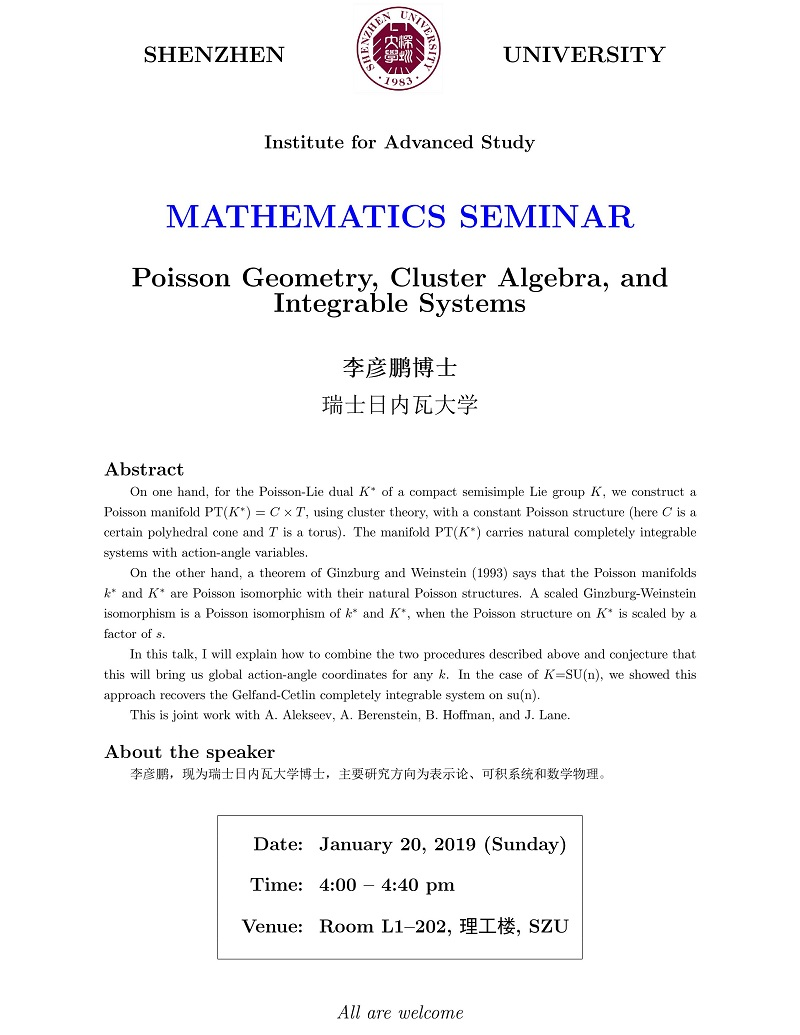﻿ 学术讲座——Poisson Geometry, Cluster Algebra, and Integrable Systems-深圳大学高等研究院

# 学术讲座——Poisson Geometry, Cluster Algebra, and Integrable Systems

On one hand, for the Poisson-Lie dual K* of a compact semisimple Lie group K, we construct a Poisson manifold PT(K*) = C×T, using cluster theory, with a constant Poisson structure (here C isa certain polyhedral cone and T is a torus). The manifold PT(K*) carries natural completely integrable systems with action-angle variables.
On the other hand, a theorem of Ginzburg and Weinstein (1993) says that the Poisson manifolds k* and K* are Poisson isomorphic with their natural Poisson structures. A scaled Ginzburg-Weinstein isomorphism is a Poisson isomorphism of k^* and K*, when the Poisson structure on K* is scaled by a factor of s.
In this talk, I will explain how to combine the two proceduresdescribed above and conjecture that this will bring us global action-angle coordinates for any k.  In the case of K=SU(n), we showed this approach recovers the Gelfand-Cetlin completely integrable system on su(n).
This is joint work with A. Alekseev, A. Berenstein, B. Hoffman, and J. Lane.